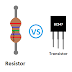The main difference between a Resistor and a Transistor is Resistor is a passive component that opposes the flow of electric current whereas a Transistor is an Active component that is used to switch or amplify electrical or electronic signals. Resistors and transistors both are very important components in electrical and electronics engineering. As we know there are a lot of differences between their construction, function, and working principle. So, we will discuss all of them in a detailed explanation.

## What is a Resistor?

A resistor is a passive electrical or electronic component that opposes the flow of electric current and converts electrical energy into heat energy. A conventional resistor has two terminal components with no polarity. Basically, they are used to reduce the current flow in a circuit. All the objects or materials in this world have their own internal resistance. In fact, a conductor also has some resistance but it is very low. Practically there is no superconductor that has zero resistance. According to this resistance, materials are divided into three types - Conductor, Insulator, and Semiconductor.

The resistor is the key element for every electrical and electronic circuit. A resistor can drop the voltage and this voltage drop depends on the value of the resistance and current flow through the resistor. For example, if a resistor of 2ohm conducts 2-ampere current then the voltage drop will be 2X2 = 4 volt. So, when a resistor is connected in series with a power source you will get a lower voltage than the actual voltage due to the voltage drop by the resistor.

## What is a Transistor?

A transistor is an active electronic component made of semiconductor material and it is used to switch or amplify the electrical or electronic signal. A transistor is a three-terminal device. Yes, a transistor can be called a device also. Here we need to apply a voltage or current to its input circuit and according to it control the current to its output circuit. The transistor is mostly used in electronic circuits, especially in digital electronic circuits. Without transistors, you may not find any digital circuit.

A transistor can be used as an amplifier where, by applying a low-power signal we can get a high-power signal, of course, here we need an auxiliary power supply also. Transistors are available individually and in the form of an integrated circuit also where multiple transistors are fabricated. Transistors work with either voltage or current or both voltage and current. The Bipolar Junction Transistor(BJT) is current-controlled device whereas the Field Effect Transistor(FET) is a voltage-controlled device.

## Difference between Resistor and Transistor

 Resistor Transistor The resistor is a Passive Component The transistor is an Active Component It resists the flow of electric current It switches or amplifies the electrical signals It converts the electrical energy into heat energy It  does not convert the energy It makes a huge power loss It creates a very low power loss Resistor makes voltage Drop Ideally, it does not make any power loss. There is no biasing required for the resistor. Biasing is required for the transistor. Act well with both AC and DC power supply. It performs well with a DC power supply.

Resistor VS Transistor | The Main DifferencesReviewed by Author on October 29, 2022 Rating: 5